# INDUCTIVE EFFECT - DEFINTION - TYPES - POSITIVE - NEGATIVE - EXAMPLES - APPLICATIONS

The polarization of a σ bond due to electron withdrawing or electron donating effect of adjacent groups or atoms is referred to as inductive effect.

A sigma bond between two atoms, which differ in their electronegativity, is polarized due to displacement of bond pair towards the more electronegative atom. In this process, the electronegative atom gets partial negative charge while the other atom gets partial positive charge. Thus induced polarity is transmitted through the sigma bonds in the molecule by creating a permanent dipole. This phenomenon is referred to as inductive effect.

It is represented by an arrow pointing towards the more electronegative atom carrying partial negative charge.### Salient features of inductive effect

* It arises due to electronegativity difference between two atoms forming a sigma bond.

* It is transmitted through the sigma bonds. No pi bonds are involved.

* The magnitude of inductive effect decreases while moving away from the groups causing it.

* It is a permanent effect. It may create permanent dipole in the molecule.

* In general, the inductive effect is relatively weak and is overshadowed by other electronic effects like resonance effect, hyperconjugation etc.

* It influences the chemical and physical properties of compounds.

## EXAMPLE OF INDUCTIVE EFFECT

The C-Cl bond in the butyl chloride, CH3-CH2-CH2-CH2-Cl is polarized due to electronegativity difference. The electrons are withdrawn by the chlorine atom. Thus the first carbon atom gets partial positive charge. In turn, this carbon atom drags electron density partially from the next carbon, which also gets partial positive charge. This will continue further and is how the inductive effect is transmitted through the carbon chain.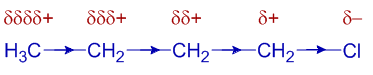One must note that the inductive effect weakens away along the chain and is not that much significant beyond the 3rd carbon atom.

Also note that inductive effect is a permanent effect and is inherent to the molecule, while the electromeric effect is a temporary effect is only created in the presence of attacking electrophilic or nucleophilic reagents.

## TYPES OF INDUCTIVE EFFECT

The inductive effect is divided into two types based on the electron withdrawing or electron releasing nature of atom/group inducing it. The strength of inductive effect is measured by comparing with that of hydrogen.

### 1) Negative inductive effect (-I):

The electron withdrawing nature of groups or atoms is called as negative inductive effect. It is indicated by -I. Following are the examples of groups in the decreasing order of their -I effect:

NH3+ > NO2 > CN > SO3H > CHO > CO > COOH > COCl > CONH2 > F > Cl > Br > I > OH > OR > NH2 > C6H5 > H

### 2) Positive inductive effect (+I):

It refers to the electron releasing nature of the groups or atoms and is denoted by +I. Following are the examples of groups in the decreasing order of their +I effect.

C(CH3)3 > CH(CH3)2 > CH2CH3 > CH3 > H

Why alkyl groups are showing positive inductive effect?

Though the C-H bond is practically considered as non-polar, there is partial positive charge on hydrogen atom and partial negative charge on carbon atom. Therefore each hydrogen atom acts as electron donating group. This cumulative donation turns the alkyl moiety into an electron donating group.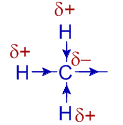## APPLICATIONS OF INDUCTIVE EFFECT

### Stability of carbocations (carbonium ions):

The stability of carbocations increase when +I groups like alkyl groups are present adjacent to positively charged carbon. The +I groups reduce the positive charge on the carbon by donating negative charge density through positive inductive effect. This results in greater stability of carbocation.

Whereas, the -I groups destabilize the carbocations as they increase the positive charge by withdrawing electron density.

Note that any factor that increases the charge (either negative or positive) on an ion results in destabilization while any factor that reduces the charge results in stabilization of that ion.

For example, the order of stability of a few carbocations containing alkyl groups is as follows:The tertiary carbocation containing three alkyl groups is more stable than the secondary carbocation with two alkyl groups and which in turn is more stable than the primary carbocation. Methyl carbocation is the least stable among the given.

In the same way the stability of free radicals increases with increase in the number of alkyl groups.

Thus the stability of different free radicals is: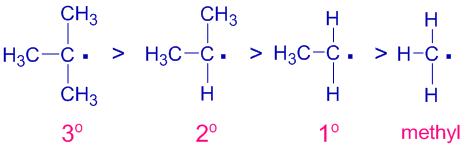### Stability of carbanions:

However, the +I groups decrease the stability of carbanions while the -I groups increases their stability.

For example, the alkyl groups (+I) donate electron density to the negatively charged carbon resulting in destabilization of carbanion.

Thus the order of stability of following carbanions is: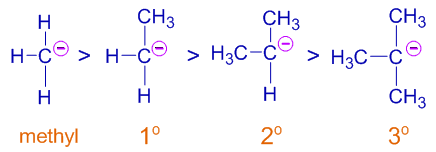Now the order is reversed. The tertiary carbanion is least stable while the methyl carbanion is most stable among the given.

### Acidic strength of carboxylic acids and phenols:

A protonic acid is always in equilibrium with its conjugate base that is formed by loss of a proton. Any factor that stabilizes the negatively charged conjugate base favors greater ionization of acid. i.e increases the acidic strength.

The electron withdrawing groups showing negative inductive effect (-I) reduce the negative charge on the conjugate base (like carboxylate ion) by pulling the electron density and thus by stabilizing it. Hence the acidic strength increases when -I groups are present.

On the contrary, the +I groups decrease the acidic strength as they destabilize the conjugate base of acid (anionic) by releasing electron density which in turn increases the negative charge on the anion and thus destabilizing it.

E.g.

i) The acidic strength increases with increase in the number of electron withdrawing Fluorine atoms as shown below.

CH3COOH < CH2FCOOH < CHF2COOH < CF3COOH

ii) Formic acid is stronger acid than acetic acid since the –CH3 group destabilizes the carboxylate ion.

On the same lines, the acidic strength of phenols increases when -I groups are present on the ring.

E.g. The p-nitrophenol is stronger acid than phenol since the -NO2 group is a -I group that withdraws electron density. Whereas, the para-cresol is weaker acid than phenol, since the -CH3 group shows positive inductive effect (+I).

For example, the order of acidic strength following phenols is: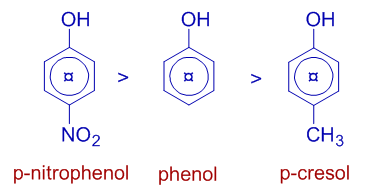Basic strength of amines:

The electron donating groups like alkyl groups increase the basic strength of amines whereas the electron withdrawing groups like aryl groups decrease the basic nature. Therefore alkyl amines are stronger Lewis bases than ammonia, whereas aryl amines are weaker than ammonia.

Thus the order of basic strength of alkyl and aryl amines with respect to ammonia is :CH3NH2 > NH3 > C6H5NH2

### Reactivity of carbonyl compounds:

The +I groups increase the electron density at carbonyl carbon. Hence their reactivity towards nucleophiles decreases. Thus formaldehyde is more reactive than acetaldehyde and acetone towards nucleophilic addition reactions.

Thus the order of reactivity follows:Question: Which of the following is an application of inductive effect?

1) Stability of carbocations

2) Basic strength of amines

3) Acidic strength of carboxylic acids

4) All of above

## Inductive effect vs Resonance effect

In general, the inductive effect is less influencing than other effects like resonance effect and hyperconjugation

E.g. The electron withdrawing nature of nitro group, -NO2 is mostly due to resonance effect rather than the inductive effect.

But there are exceptions. For example, in the cases of halogens, the negative inductive effect is more dominating than positive resonance effect.

## INDUCTIVE EFFECT MCQ QUESTIONS & ANSWERS- IIT JEE NEET IIT JAM CSIR NET CHEMISTRY

Question-1) The inductive effect can be best described as:

a) the conjugation of σ-bonding orbital with the adjacent π-orbital.

b) the ability of atom or group to cause bond polarization

c) the transfer of lone pair of electrons from more electronegative atom to lesser electronegative atom in a molecule.

d) All of the above.

Question-2) Which of the following statement is incorrect about the inductive effect ?

a) It is a permanent effect

b) It decreases with increase in distance from the polar group causing inductive effect.

c) It involves delocalization of π-electrons

d) It involves displacement of σ-electrons

Question-3) Which of the following shows positive (+I) inductive effect?

a) – NO2

b) –OCH3

c) –COOH

d) –CH

Question-4) Which of the following group shows negative (-I) inductive effect ?

a) –CH2CH

b) –C(CH3)3

c) –C6H5

d) –CH3

Question-5) Which of the following orders is correct regarding the -I effect of the substituents ?

a) –NH2< –OR > –F

b) -CR3 < –NR2< –OR < –F

c) -CR3 > –NR2> –OR > –F

d) –+NR3 > –+OR2

6) The correct order with respect to the magnitude of negative inductive effect for the given groups is:

a) –SO3H > –SO2R > –SR > –SOR

b) –SR > –SO2R > – SOR > –SO3H

c) –SO3H > –SO2R > –SOR > –SR

d) –SR > –SOR > –SO2R > –SO3H

Question-7) The most stable carbonium ion among the following is:

a) CH3+   b) CH3CH2+   c) (CH3)2CH+   d) (CH3)3C+

Question-8) The least stable radical among the following is:

a) CH3•   b) CH3CH2•   c) (CH3)CH•   d) (CH3)3C•

Question-9) The correct order of acidic strength of given acids is:

a) Formic acid > Acetic acid > Propanoic acid

b) Formic acid > Acetic acid < Propanoic acid

c) Formic acid < Acetic acid > Propanoic acid

d) Formic acid < Acetic acid < Propanoic acid

Question-10) The correct order of acidic strength is:

a) CH3CH2CHFCOOH > CH3CHFCH2COOH > FCH2CH2CH2COOH

b) CH3CH2CHFCOOH < CH3CHFCH2COOH < FCH2CH2CH2COOH

c) CH3CH2CHFCOOH > CH3CHFCH2COOH < FCH2CH2CH2COOH

d) CH3CH2CHFCOOH < CH3CHFCH2COOH > FCH2CH2CH2COOH

Hint: The strength of inductive effect depends on number of fluorines and their distance from the -COOH group.

Question-11) Which of the following is strongest acid ?

a) Cl3CCOOH

b) Cl2CHCOOH

c) ClCH2COOH

d) CH3COOH

Question-12) Which of the following carbanion is more stable ?

a) CH3- b) CH3CH2- c) CF3CH2- d) (CH3)2CH-

Question-13) The correct order of acidic strength of following substituted phenols is :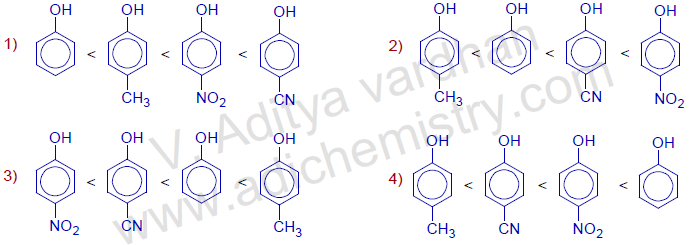Question-14) What is the %s character in hybridization of carbon when it shows strongest -I effect ?

a) 25% b) 50% c) 75% d) 66.6%

Information: The electronegativity of carbon increases with the % of s-orbital character in the hybridization. Thus the order of electronegativity is  sp >sp2 > sp3. Therefore the carbon with sp hybridization (as in alkynes) shows greatest inductive effect.

Question-15) Which of the following orders is correct regarding the acidity of carboxylic acids ?

a) (CH3)3CCOOH > (CH3)2CHCOOH > CH3CH2COOH

b) (CH3)3CCOOH > (CH3)2CHCOOH < CH3CH2COOH

c) (CH3)3CCOOH < (CH3)2CHCOOH > CH3CH2COOH

d) (CH3)3CCOOH < (CH3)2CHCOOH < CH3CH2COOH

Question-16) The strongest Lewis base among the following is :

a) CH3NH2

b) (CH3)2NH

c) (CH3)3N

d) C6H5NH2

Question-17) Identify the correct statements about the electronegativity of groups given below:  (CSIR NET JUNE 2018)

(A) CF3 group has greater value than that of NF2

(B) NH2 group has lower value than that of NF2

(C) OH group has greater value than that of NF2

(D) CH3 and C2H5 groups have almost similar values

1) A, B and D

2) B and C

3) B, C and D

4) B and D

Question-18) Which of the following is an incorrect statement about inductive effect?

A) The electron-withdrawing inductive effect (-I) of a group weakens the O-H bond in -COOH and favours ionisation of a carboxylic acid

B) It operates through space.

C) Aniline is a weaker base than ammonia due to negative inductive effect shown by the phenyl group.

D) Alkyl groups are good examples of positive inductive effect.

Question-19) Choose the correct statement regarding inductive effect.

a) It is strengthened with increase in distance from the atom or group causing polarization.

b) Involves displacement of a lone pair.

c) Involves delocalization of pi electrons.

d) It operates through σ-bonds.

Question-20) Which of the following groups has highest inductive effect.

a) Cl

b) Br

c) F

d) I

Question-21) Which of the following statements about inductive effect is correct?

a) It is also known as hyperconjugation.

b) Magnitude of inductive effect increases with increase in the distance from group causing polarization.

c) It involves delocalization of π-electrons

d) It involves displacement of σ-electrons

Question-22) Which of the following group shows electron withdrawing inductive effect ?

a) –CH2CH

b) – NO2

c) –C6H5

d) –CH3

Question-23) inductive effect in molecule arises due to :

a) presence of a non-polar bond

b) polarization of a sigma bond adjacent to either an electron withdrawing or electron releasing group.

c) delocalization of π-electrons

d) delocalization of sigma electrons.

Question-24) Which of the following effect can destabilize the 3o butyl carboanion? :

a) positive inductive effect

b) negative inductive effect.

c) neutral inductive effect

d) None of the above.